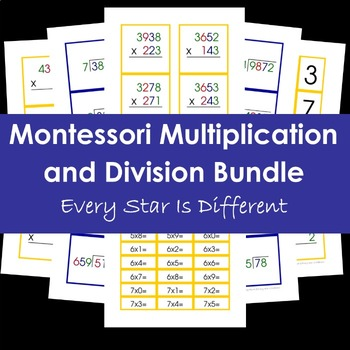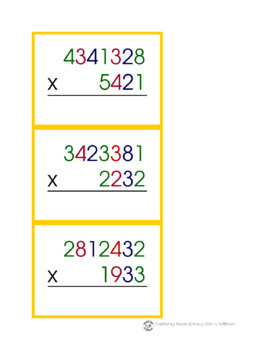DID YOU KNOW:
Seamlessly assign resources as digital activities

Learn how in 5 minutes with a tutorial resource. Try it Now

Learn More# Montessori Multiplication and Division BundleGrade Levels
PreK - 5th
Subjects
Resource Type
Formats Included
• PDF
Pages
273 pages

### Description

This bundle helps your child progress through the Montessori preschool and elementary curriculums. It includes simple multiplication and division facts all the way through divison problems with divisors up to three digits and answers with remainders.

Multiplication and Division concepts are broken down into the smallest steps, with fifty equation cards for each step. This is so helpful for large classroom settings, a child who needs extra practice, and those who just can't get enough math problems to solve.

Cards are created using the Montessori place value colors to reinforce concepts already learned, to help children keep their work organized, and to provide extra visual cues and support for those who may need it.

The Montessori Multiplication and Division Bundle is your one stop resource for all of your home and classroom needs!

Just take a look at this table of contents!

Multiplication

-Numbers and Signs for Multiplication

-Multiplication Strips

-50 Static Multiplication Equation Cards to 9,999 multiplied by one digit numbers

-50 Static Multiplication Equation Cards to 9,999 multiplied by two, three, and four digit numbers

-50 Dynamic Multiplication Equation Cards to 9,999 multiplied by one digit numbers

-50 Dyn-amic Multiplication Equation Cards multiplied by one digit numbers and answers to 99,999

-50 Dynamic Multiplication Equation Cards to 9,999 multiplied by two, three and four digits

-50 Static Multiplication Equation Cards to 9,999,999 multiplied by one digit numbers

-50 Static Multiplication Equation Cards to 9,999,999 multiplied by two, three, and four digit numbers

-50 Dynamic Multiplication Equation Cards to 9,999,999 multiplied by one digit numbers

-50 Dynamic Multiplication Equation Cards to 9,999,999 multiplied by two, three, and four digit numbers

Division

-Numbers and Signs for Division

-Division Strips

-50 Long Division with four digit dividends and one digit divisors, no remainders

-50 Long Division with four digit dividends and two digit divisors, no remainders

-50 Long Division with two digit dividends and one digit divisors, remainders

-50 Long Division with four digit dividends and one digit divisors, remainders

-50 Long Division with four digit dividends and two digit divisors, remainders

-50 Long Division with five, six, and seven digit dividends and one digit divisors, no remainders

-50 Long Division with five, six and seven digit dividends and one digit divisors, remainders

-50 Long Division with five and six digit dividends and two digit divisors, no remainders

-50 Long Division with five and six digit dividends and two digit divisors, remainders

-50 Long Division with five an six digit dividends and three digit divisors, no remainders

-50 Long Division with five and six digit dividends and three digit divisors, remainders

-Large Graph Paper

-Small Graph Paper

Total Pages
273 pages
Answer Key
N/A
Teaching Duration
N/A
Report this Resource to TpT
Reported resources will be reviewed by our team. Report this resource to let us know if this resource violates TpT’s content guidelines.

### Questions & Answers

Teachers Pay Teachers is an online marketplace where teachers buy and sell original educational materials.

More About Us